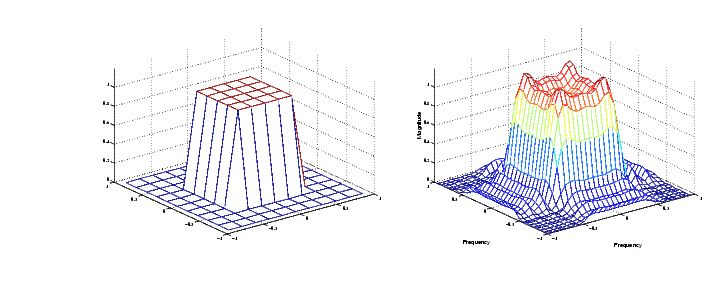Image Processing Toolbox User's GuideFrequency Sampling Method

The frequency sampling method creates a filter based on a desired frequency response. Given a matrix of points that define the shape of the frequency response, this method creates a filter whose frequency response passes through those points. Frequency sampling places no constraints on the behavior of the frequency response between the given points; usually, the response ripples in these areas. (Ripples are oscillations around a constant value. The frequency response of a practical filter often has ripples where the frequency response of an ideal filter is flat.)

The toolbox function `fsamp2` implements frequency sampling design for two-dimensional FIR filters. `fsamp2` returns a filter `h` with a frequency response that passes through the points in the input matrix `Hd`. The example below creates an 11-by-11 filter using `fsamp2` and plots the frequency response of the resulting filter. (The `freqz2` function in this example calculates the two-dimensional frequency response of a filter. See Computing the Frequency Response of a Filter for more information.)

• ```Hd = zeros(11,11); Hd(4:8,4:8) = 1;
[f1,f2] = freqspace(11,'meshgrid');
mesh(f1,f2,Hd), axis([-1 1 -1 1 0 1.2]), colormap(jet(64))
h = fsamp2(Hd);
figure, freqz2(h,[32 32]), axis([-1 1 -1 1 0 1.2])```

Desired Two-Dimensional Frequency Response (left) and Actual Two-Dimensional Frequency Response (right)

Notice the ripples in the actual frequency response, compared to the desired frequency response. These ripples are a fundamental problem with the frequency sampling design method. They occur wherever there are sharp transitions in the desired response.

You can reduce the spatial extent of the ripples by using a larger filter. However, a larger filter does not reduce the height of the ripples, and requires more computation time for filtering. To achieve a smoother approximation to the desired frequency response, consider using the frequency transformation method or the windowing method.Frequency Transformation Method Windowing Method